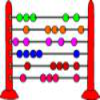#### You may also like### Which Scripts?

There are six numbers written in five different scripts. Can you sort out which is which?### A Romantic Riddle

Read this riddle and see if you can work out how the trees must be planted.### Fibonacci's Three Wishes 1

First of two articles about Fibonacci, written for students.

# Roman Numerals

## Roman Numerals

These symbols are the building blocks of Roman numerals:

I, V, X, L, C, D and M

Do you know the value of each letter?  Click on 'Show' to check...

I = 1
V = 5
X = 10
L = 50
C = 100
D = 500
M = 1000

In our number system (the Arabic numeral system), there are ten different digits, (0, 1, 2 , 3, 4, 5, 6, 7, 8, 9) and the place of these digits in the number determines its value. For example, 2 on its own means 'two', but in 3240, the '2' now means "two hundred". In this way, any number can be written down, using only ten digits.

Roman numerals have a set of rules which allow you to write down any number:

1. If a smaller numeral comes after a larger numeral, add the smaller number to the larger number;
2. If a smaller numeral comes before a larger numeral, subtract the smaller number from the larger number;
3. Do not use the same symbol more than three times in a row.

Can you use these rules to construct and decipher Roman numerals?
Try converting the following Roman numerals into Arabic numerals:

III
IV
XVIII
XIX
MCMLXXVI
MMXXIII
MCMLXII

Now try converting the following into Roman numerals:

55
86
two thousand five hundred and ninety-two
913

Can you work out how to write '1984' in Roman numerals?

'1984' written in Roman numerals has more numerals than when it is written in Arabic numerals. However, this will not always be the case.

Can you find some examples when the number of Roman numerals is fewer than the number of Arabic numerals for the same number?

### Why do this problem?

This activity introduces children to Roman numerals.  Comparing the number of numerals used in the two different systems makes an interesting context in which to practise interpreting and creating Roman numerals.

### Possible approach

You could begin by inviting learners to share anything they know already about Roman numerals.

Challenge learners to decipher the Roman numerals given (perhaps in pairs) and use these examples to draw out the three rules. You could then invite pairs to create the Roman numerals for the numbers written in Arabic numerals in the problem, which will help them familiarise themselves with the rules.

The group can then work on the task of finding numbers which use fewer numerals in the Roman numeral system than the Arabic system.

### Key questions

How did you work this one out?
What would you say are the differences between Roman numerals and the ways we write numbers now?

### Possible extension

Learners might enjoy investigating which Roman numerals read the same backwards as forwards.